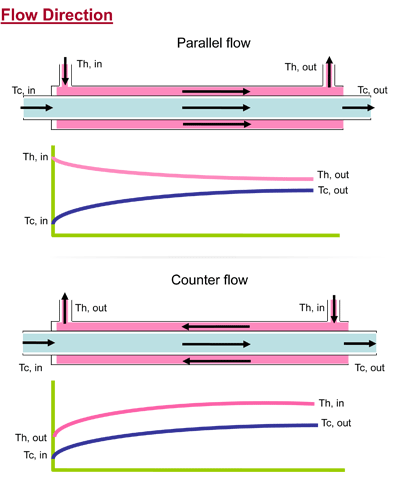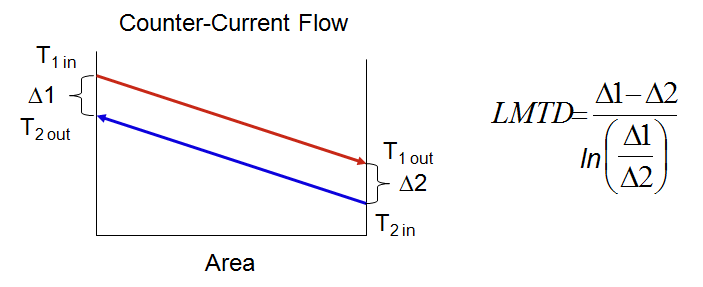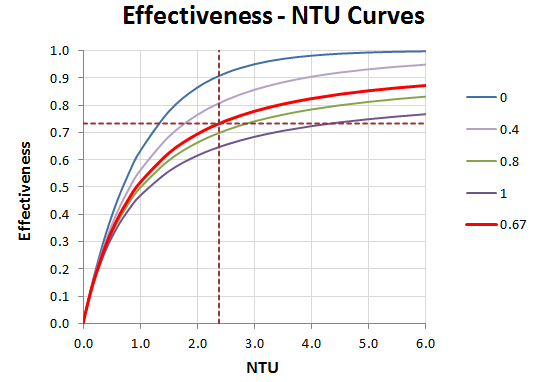More
HomeScienceHow to Calculate the Efficiency of Heat Exchangers?

# How to Calculate the Efficiency of Heat Exchangers?

Table of Contents

The term efficiency of heat exchanger is not defined as a unique concept in scientific resources. However, to determine the efficiency of any system, an ideal state is considered, based on which the degree of proximity of the system to that state is defined as efficiency. In the case of a heat exchanger, the ideal state is not definitively determined. But methods are provided for this purpose, which we will discuss below.

## Analysis of Heat Exchanger

There are generally two ways to evaluate the performance of heat exchangers. One is Log Mean Temperature Difference, abbreviated as LMTD, and the other is the Effectiveness NTU-method, denoted by ε-NTU.

Meanwhile, by defining the efficiency of heat exchangers, another general method can be considered as a method for analyzing the performance of heat exchangers. This criterion shows how far the heat exchanger is from its best and most ideal design point and how much change it needs to reach this desired state.

Read More on Linquip

### Definition of Efficiency of Heat Exchanger

The heat exchange process between two fluids with different temperatures using solid walls occurs in various engineering applications. The tool to achieve this exchange is a heat exchanger. Some applications like air conditioning, power generation, waste heat recovery, and chemical processing use this device.

The basis of the work of a heat exchanger is that the hot fluid enters the heat exchanger at temperature T1 and its heat capacity is Chot. Also, the cold fluid with the heat capacity of Ccold enters temperature t1; in the meantime, the hot fluid loses its heat, and its temperature drops to T2. It delivers heat to the cold fluid to increase its temperature to t2 and leave the heat exchanger at this temperature.A simple schematic of a heat exchanger (Reference: energyeducation.ca)

In general, the efficiency of heat exchange is defined as the ratio of the amount of heat transferred in the actual case to the amount of heat in the ideal case, in the optimal case (equation below).

\eta =\frac{q_{act}}{q_{ideal}}

The heat transfer rate in the actual state is defined as the basis of the rate of change of cold or hot fluid temperature.

q_{act}=C_{hot}\left(T_1-T_2\right)

Or

q_{act}=C_{cold}\left(t_2-t_1\right)

Also, the optimal heat transfer can be calculated as the following equation.

q_{ideal}=UA\left(\overline{T}-\overline{t}\right)

We denote the surface at which heat exchange takes place by A. Also, the overall average heat transfer coefficient is equal to U. The phrase in parentheses is called the Arithmetic Mean Temperature Difference (abbreviated as AMTD) and is calculated as follows.

\overline{T}-\overline{t}\mathrm{=}\frac{T_1+T_2}{2}-\frac{t_1+t_2}{2}

The best heat transfer occurs in the case of the counter flow heat exchanger.

Also, to find the overall average heat transfer coefficient (U) we can use the following relationship.

\frac{1}{UA}=\frac{1}{U_{hot}A_{hot}}=\frac{1}{U_{cold}A_{cold}}

A general equation can be used to calculate the efficiency of heat exchanger developed for a number of known exchangers.

\eta =\frac{{\mathrm{tanh} \left(Fa\right)\ }}{Fa}

In the equation above, Fa is the fin analogy number which can be evaluated for some common heat exchanger as what follows. In the following section, we refer to counter flow and parallel flow heat exchangers.

#### Counter Flow Heat Exchanger

The direction of flow of hot and cold fluids in counter flow converters is the opposite. In this can we have the following relation to calculate Fa.

Fa=NTU\frac{(1-C_r)}{2}

In this equation, NTU is the number of transfer units and is equal to:

NTU=\frac{UA}{C_{min}}

C{_{min}}=min(C{_{hot}},C{_{cold}})

Also, Cr is the capacity ratio and defined as:

C_r=\frac{C_{min}}{C_{max}}

#### Parallel Flow Heat Exchanger

In a parallel flow heat exchanger, hot and cold fluids move in the same direction. To find Fa the following relation is suggested.

Fa=NTU\frac{(1+C_r)}{2}Comparison between the structure of parallel flow and counter flow heat exchangers (Reference: quora.com)

### Evaluating the Heat Exchanger Using LMTD Method

Consider q is the rate of heat transfer within a heat exchanger between the hot and cold fluids. Because heat transfer between the exchanger and its surroundings is negligible, we rewrite the transferred heat based on the parameters we defined in previous parts as following.

q=C_{hot}\left(T_1-T_2\right)=C_{cold}\left(t_2-t_1\right)

The temperatures in the equations point to the mean fluid temperatures at the designated sections.

To write the heat transfer using the overall heat transfer coefficient, we consider another expression as follows.

\mathrm{\Delta }\mathrm{T}=T_{hot}-T_{cold}

Since ΔT varies with location through the heat exchanger, it is important to deal with an equation in the form of:

q=UA\mathrm{\Delta }T_m

ΔTm is mean temperature difference. Now, we need to establish an expression for this parameter. It is shown in the references that the appropriate temperature difference should be expressed based on the logarithmic mean temperature difference, which is defined as follows.

\mathrm{\Delta }T_m=\mathrm{\Delta }T_{lm}=\frac{\mathrm{\Delta }T_1-\mathrm{\Delta }T_2}{{\mathrm{ln} \left(\frac{\mathrm{\Delta }T_1}{\mathrm{\Delta }T_2}\right)\ }}=\frac{\mathrm{\Delta }T_2-\mathrm{\Delta }T_1}{{\mathrm{ln} \left(\frac{\mathrm{\Delta }T_2}{\mathrm{\Delta }T_1}\right)\ }}

Thus,

q=UA\mathrm{\Delta }T_{lm}

In the definition of logarithmic mean temperature difference (LMTD), indices 1 and 2 are related to the sections from which entry or exit occurs. This means that one of the following two conditions happens.

#### Parallel Flow Heat Exchanger

In this type of heat exchangers, as two fluids enter and exit in the same directions, we have:

\mathrm{\Delta }T_1=T_1-t_1

\mathrm{\Delta }T_2=T_2-t_2

#### Counter Flow Heat Exchanger

Since in counter flow heat exchangers, hot and cold fluid flows enter or leave in opposite directions:

\mathrm{\Delta }T_1=T_1-t_2

\mathrm{\Delta }T_2=T_2-t_1

Based on these equations, the calculation of the actual heat transfer can be performed.How to calculate temperature differences in the LMTD method for a counter flow heat exchanger (Reference: virginiaheattransfer.com)

### Evaluating the Heat Exchanger Using ε-NTU Method

Based on this method, the effectiveness of the exchanger is defined. Of course, it is necessary to determine the maximum allowable heat transfer rate from a heat exchanger. This amount can be achieved by a counter flow heat exchanger of infinite length. In such an exchanger, one of the two fluids will have the maximum allowable temperature change; that’s mean:

{\mathrm{\Delta }T}_{max}=T_1-t_1

The first case is to assume Cc<Ch. Then we have:

q_{max}=C_c\left(T_1-t_1\right)

And if Cc>Ch, the maximum allowable heat transfer is:

q_{max}=C_h\left(T_1-t_1\right)

After summarizing the last two relationships, we can write:

q_{max}=C_{min}\left(T_1-t_1\right)

C_{min}={\mathrm{min} \left\{C_c,C_h\right\}\ }

The efficiency of the heat exchanger or its effectiveness can now be defined as follows:

\varepsilon =\frac{q}{q_{max}}

It can be shown for each heat exchanger:

\varepsilon =f\left(NTU,\ C_r\right)

Both NTU and Cr are introduced in the previous sections. There are some relations developed to calculate the function mentioned above. Each expression belongs to a specific class of exchangers. In the following, some of these classes and the related equations are presented.

#### Parallel Flow Heat Exchanger

Efficiency of heat exchanger with the parallel arrangement is:

\varepsilon =\frac{1-{\mathrm{exp} [-NTU\left(1+C_r\right)]\ }}{1+C_r}

#### Counter Flow Heat Exchanger

Efficiency of heat exchanger with the parallel pattern is:

(C­r<1)

\varepsilon =\frac{1-{\mathrm{exp} [-NTU\left(1-C_r\right)]\ }}{1-C_r{\mathrm{exp} [-NTU\left(1-C_r\right)]\ }}

(C­r=1)

\varepsilon =\frac{NTU}{1+NTU}

#### Shell and Tube Heat Exchanger

Efficiency of heat exchanger with the shell and tube structure (n shell passes) is:

\varepsilon =\left[{\left(\frac{1-{\varepsilon }_1C_r}{1-{\varepsilon }_1}\right)}^n-1\right]-\left[{\left(\frac{1-{\varepsilon }_1C_r}{1-{\varepsilon }_1}\right)}^n-C_r\right]

Where ε1 is the efficiency of heat exchanger with one pass shell and tube structure.

{\varepsilon }_1=2{\left[1+C_r+{\left(1+C^2_r\right)}^{\frac{1}{2}}\times \frac{1+{\mathrm{exp} {\left[-(NTU\right)}_1{\left(1+C^2_r\right)}^{\frac{1}{2}}]\ \ }}{1-{\mathrm{exp} {\left[-(NTU\right)}_1{\left(1+C^2_r\right)}^{\frac{1}{2}}]\ \ }}\right]}^{-1}

#### Cross Flow Heat Exchanger

To calculate the efficiency of heat exchanger with cross flow formation, usually, a correction factor is regarded for the logarithmic mean temperature difference. This means:

\mathrm{\Delta }T_{lm}=F\mathrm{\Delta }T_{lm.counter\ flow}

The correction factor is determined using diagrams for different types of heat exchangers.

In the following figure, you can see the curves to find the effectiveness of a cross flow heat exchanger for different values of NTU and Cr.Effectiveness-NTU curves for different values of Cr (Reference: cheguide.com)

#### Heat Exchangers with Cr=0

The efficiency of heat exchanger is calculated with the following equation.

\varepsilon =1-{\mathrm{exp} \left(-NTU\right)\ }

## Conclusion

In this article, we reviewed the efficiency of heat exchanger, explained the methods introduced to calculate this parameter, and mentioned the equations for different types of heat exchangers.

Recent Articles

### Steam Turbine Efficiency: Complete Explanation

#### 1 Comment

1.Mehmet ŞİRİN

We studied this topic in the heat transfer course at the university.

0Shares